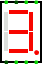#7-Segment Display

 Library: Input/Output Introduced: 2.1.3 Appearance:## Behavior

Displays the values of its eight one-bit inputs. Segments are either colored or light gray depending on the inputs. The correspondence is as follows.(Manufacturers vary as to how they map inputs to segments; the correspondence used here is based on Texas Instruments' TIL321.)

## Pins

North edge, first from left (input, bit width 1)
Controls the middle horizontal segment.
North edge, second from left (input, bit width 1)
Controls the upper vertical segment on the left side.
North edge, third from left (input, bit width 1)
Controls the upper horizontal segment.
North edge, fourth from left (input, bit width 1)
Controls the upper vertical segment on the right side.
South edge, first from left (input, bit width 1)
Controls the lower vertical segment on the left side.
South edge, second from left (input, bit width 1)
Controls the bottom horizontal segment.
South edge, third from left (input, bit width 1)
Controls the lower vertical segment on the right side.
South edge, fourth from left (input, bit width 1)
Controls the decimal point.

## Attributes

On Color
The color with which to draw the display segments and decimal point when they are on.
Off Color
The color with which to draw the display segments and decimal point when they are off.
Background
The color with which to draw the display's background (transparent by default).
Active On High?
If yes, then the segments light when the corresponding input is 1. If no, they light when the corresponding input is 0.

None.

## Text Tool Behavior

None.

Back to Library Reference